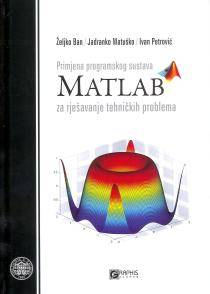### Laboratory and Skills - Matlab

#### Course Description

The purpose of this course is to provide the students with a working introduction to the MATLAB technical computing environment and give the practical knowledge about programming techniques in MATLAB. Themes of vector and matrix data analysis, graphical visualization, data modeling, and MATLAB programming are explored in the context of realistic examples.

#### General Competencies

The students will get acquainted with MATLAB 7.X user interface, be able to work with MATLAB variables, interactively process data, plot and visualizes results, create and debug M-files and build graphical user interface.

#### Learning Outcomes

1. list basic Matlab comands
2. describe the method of the problem solvin using Matlab
3. explain the description of dynamic systems in Matlab
4. use Matlab for simulation of the dinamic systems
5. analyze the obtained results
6. illustrate the results using MATLAB graphics tools
7. combine different Matalab and Simulink tools for determining the behavior of dynamic systems
8. assess the validity of the results

#### Forms of Teaching

Lectures

Lectures 8 hours

Exams

Laboratory exercises - test Final exam

Laboratory Work

Two hours opf exercises per week.

#### Grading Method

Continuous Assessment Exam
Type Threshold Percent of Grade Threshold Percent of Grade
Laboratory Exercises 0 % 33.33 % 0 % 33.33 %
Final Exam: Written 0 % 66.67 %
Exam: Written 0 % 66.67 %
##### Comment:

This course has no midterm exam.

#### Week by Week Schedule

1. 1st Lecture Overview of Matlab software. Basic operations in MATLAB. Application of M functions. Graphic presentation of results in 2D and 3D space. Application of the Symbolic Toolbox.
2. 2nd Lecture Applying Simulink for simulation of the dynamical technical systems.
3. Preparing for the first Laboratory exercise (LV1) Basic operation of the program Matlab.
4. Work on the first Laboratory exercise (LV1) Basic operation of the program Matlab.
5. Finalizations of the first Laboratory exercise (LV1) and analyze of the results.
6. Preparing for the second Laboratory exercise (LV2) Design of the M scripts and M functions.
7. Work on the second Laboratory exercise (LV2). Using M scripts and M functions.
8. Finalizations of the second Laboratory exercise (LV2) and analyze of the results.
9. Preparing for the third Laboratory exercise (LV3). Preparation for solving problems using symbolic expressions.
10. Work on the third Laboratory exercise (LV3). Solving problems using Symbolic Toolbox.
11. Finalizations of the third Laboratory exercise (LV3) and analyze of the results.
12. Preparing for the fourth Laboratory exercise (LV4). Modelling of RLC circuit using Matlab / Simulink.
13. Work on the foruth Laboratory exercise (LV4). Simulation of RLC circuit using Matlab / Simulink.
14. Finalizations of the fourthLaboratory exercise (LV4) and analyze of the results.
15. Final exam.

#### Study Programmes

##### University undergraduate
Electrical Engineering and Information Technology and Computing (study)
(2. semester)

#### LiteratureŽ. Ban, J. Matuško, I. Petrović (2010.), Primjena programskog sustava Matlab za rješavanje tehničkih problema, Graphis, Zagreb
(.), MATLAB 7.x Documentation The Mathworks The Mathworks 2005,

#### General

ID 104307
Summer semester
2 ECTS
L1 English Level
L1 e-Learning

50 Acceptable